• 集算器
教程
函数参考
习题
代码参考
用户参考
外部库使用指南
数据文件工具用户参考
DQL教程
集群管理器使用说明
SPL命令执行器用户参考
• 润乾报表
教程
填报教程
分析教程
报表中心教程
用户参考
程序员参考
高级指南
通用查询
DQL部署集成手册
• 易明建模
用户参考
json参数说明
• 润乾官网

# 线性代数函数

## 向量和矩阵

 A 1 [-5, 8, 11] 2 =A1(3) 3 [[-5, 8, 11]] 4 [[1,3,5],[2,4,7]] 5 =A4(2)(1) 6 =transpose(A4)

A1中用序列直接定义向量，这种向量和显示结果相同，是单列的纵向量A 1 [[1,3,5],[2,4]] 2 =A1(2) 3 =A1.(~(2)) 4 =A1.len() 5 =A1.max(~.len())## 基本计算

 A B 1 [1,1,0,0] [1,2,3,4] 2 [[1,3,5],[2,4,7]] [[-5, 8, 11], [3, 9, 21]] 3 =A1++B1 =A1--B1 4 =A2.(~++B2(#)) =A2.(~--B2(#))

A3B3计算两个向量对位成员相加或相减的结果如下：A4B4中计算两个矩阵对位成员相加或相减的结果如下：A B 1 [1,1,0] [1,2,3] 2 =mul(A1,B1) =mul([A1],B1)

A2中用mul() 函数计算两个向量的乘积，默认情况下会计算两个向量的外积，同时计算时会采用双精度计算以保证精度。A2中计算两个3行向量的外积，会自动将第2个纵向量转置为横向量，得到的外积为3*3矩阵，如下：A B 1 [[1,3,5],[2,4,7]] [[-5, 8, 11], [3, 9, 21], [4, , 8] 2 [8, 9, 0] 3 =mul(A1,B1) =mul([A1(2)], B1.(~(2))) 4 =mul(A1, A2)mul(A, B) 的结果中，结果中第mn列的每个成员都是A矩阵中第m行与B矩阵中第n列的内积，如B3中计算第2行第2列的结果如下：A B 1 [[1, 0, 2], [-1, 5, 0], [0, 3, -9]] [[3, 2, 4], [-1, 1, 2], [9, 5, 10]] 2 =det(A1) =det(B1) 3 =rankm(A1) =rankm(B1) 4 =inverse(A1)

det() 函数可以计算矩阵行列式的值，如果矩阵不满秩，得到的结果会是0A2B2中结果如下：rankm() 函数可以计算矩阵的A3B3中得到两个矩阵的秩如下：## 数学计算

 A B 1 [4, 1, 10] [-7, 4, 7] 2 =var(A1) =var@s(A1) 3 =mse(A1, B1) =mae(A1, B1) 4 =dis(A1, B1) 5 =dis@a(A1, B1) =dis@m(A1, B1)

var() 函数可以计算向量的总体方差，如果添加@s选项则计算的是统计时常用的样本方差A2B2中结果如下：mse()mae() 函数可以计算两个向量的标准误差和绝对误差，A3B3中结果如下：dis() 函数可以计算两个向量间的欧氏距离，添加@a选项计算距离时将计算绝对值的和，添加@m选项将根据向量长度求均值。A4, A5B5中结果如下：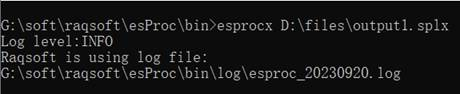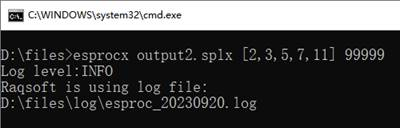pearson() 函数和spearman()函数，可以计算两个向量的皮尔逊相关系数和斯皮尔曼相关系数：

 A B 1 [4, 1, 10] [-7, 4, 7] 2 =pearson(A1, B1) =spearman(A1, B1) 3 =pearson@r(A1, B1) =pearson@a(A1, B1; 2)

A2A3中得到的pearson相关系数和spearman相关系数如下：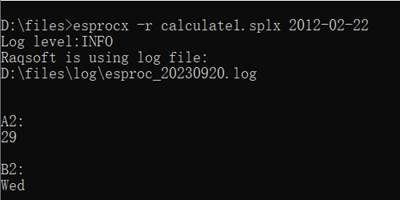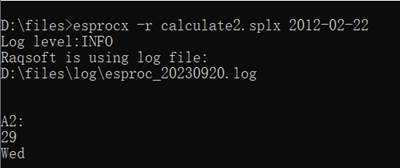A B 1 [4, 1, 10] [[8, 1, 6], [3, 5, 7], [4, 9, 2]] 2 =norm(A1) =norm@0(A1) 3 =norm@s(A1) =norm(B1)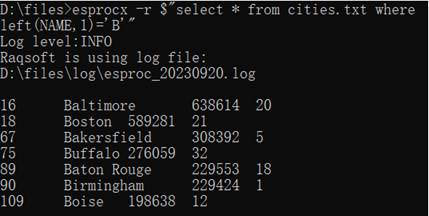linefit(A, Y) 函数可以用最小二乘法做线性拟合，其中A为系数矩阵，Y为常数矩阵，两者行数相同。当An阶方阵，Y为向量时相当于求解矩阵方程AX=Y，如：

 A B 1 [[1,-2, 3],[2,3,4],[3,5,7]] [-10,5,7] 2 =linefit(A1,B1)A B 1 [1,2,3,5] [2,13,38,155] 2 =polyfit(A1,B1,1) =polyfit(A1,B1,2) 3 =polyfit(A1,B1,3)

A2B2A3中分别使用了不同的次数来拟合两个同样的向量，计算后的结果分别如下：A3中的拟合结果即为1.041667x3+0.75x2+1.458333x-1.25